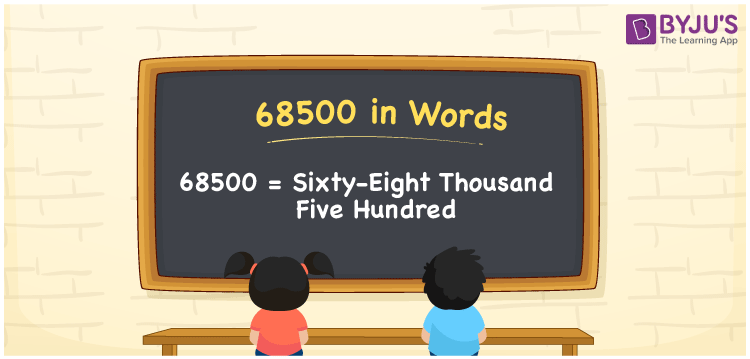# 68500 in Words

68500 in words is written as “Sixty-Eight Thousand Five Hundred”. For example, in a bank, a cheque of Rs. 68500 is given by Rupees “Sixty-Eight Thousand Five Hundred only”. In Mathematics, 68500 is a cardinal number, that is used to represent the quantity or value of something. 68500 can be used to represent the population, price of a product, or it can be used as a currency for any country such as 68500\$. Let us see in this article how to write 68500 in words using the place value method.

 68500 in Words Sixty-Eight Thousand Five Hundred Sixty-Eight Thousand Five Hundred in Numbers 68500

## 68500 in English Words## How to Write 68500 in Words?

68500 is a five-digit number. The place value of each digit in the number 68500 will help in determining the name of the number. Hence, we have a place value chart here to write the number 68500 in words.

 Ten Thousands Thousands Hundreds Tens Ones 6 8 5 0 0

We can see, from the above table, the positions of digits are:

• 6 → Ten Thousands
• 8 → Thousands
• 5 → Hundreds
• 0 → Tens
• 0 → Ones

Hence, when we read the number from right to left, it is Sixty-Eight Thousand Five Hundred. Learn more about numbers in words at BYJU’S.

### Expanded Form of 68500

We can write the expanded form as:

6 × Ten thousand + 8 × Thousand + 5 × Hundred + 0 × Ten + 0 × One

= 6 × 10000 + 8 × 1000 + 5 × 100 + 0 × 10 + 0 × 1

= 60000 + 8000 + 500 + 0 + 0

= 68500

= Sixty-Eight Thousand Five Hundred

68500 is the natural number that is succeeded by 68499 and preceded by 68501. Learn more about the number 68500 below:

• 68500 in Words – Sixty-Eight Thousand Five Hundred
• Is 68500 an odd number? – No
• Is 68500 an even number? – Yes
• Is 68500 a perfect square number? – No
• Is 68500 a perfect cube number? – No
• Is 68500 a prime number? – No
• Is 68500 a composite number? – Yes

## Frequently Asked Questions on 68500 in words

Q1

### What is 68500 in words?

68500 in words is given by Sixty-Eight Thousand Five Hundred.
Q2

### What is the place value of 5 in 68500?

The place value of 5 in 68500 is Hundreds.
Q3

### How to spell 68500 in English words?

68500 in English is spelt as Sixty-Eight Thousand Five Hundred